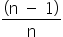Mathematics
Easy

Question

# Statement 1: Let n be some integer greater than 1, then flooris 1. Statement 2: Let n be some integer greater than 1, then ceilingis 1.

## Only statement 1 is correctOnly statement 2 is correct Both statements 1 & 2 are correct Both statements 1 & 2 are not correctHint:

## The correct answer is: Only statement 2 is correct

### We have to find which of the two given statements are true.Sincewill always be less than one, thus, flooris 0. Sincewill always be less than one, thus, ceilingis 1.Hence, the correct option is A.

The ceiling function is also known as the smallest integer function. The floor function is the greatest integer function.

### Related Questions to study#### With Turito Foundation.#### Get an Expert Advice From Turito.